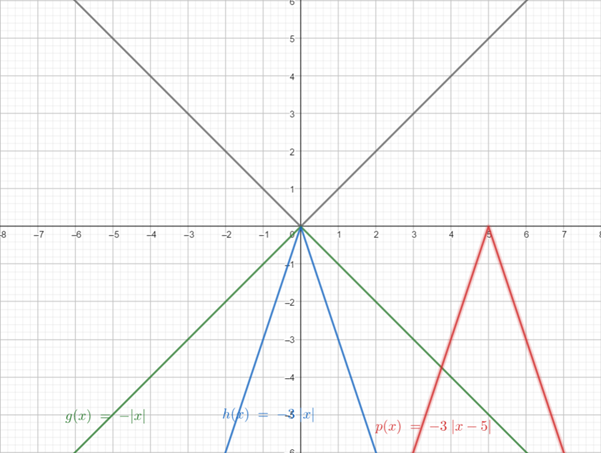# To graph the functionson the same screen and how they related to the graph in part (a). The functions are given below: ( a ) y 1 = | x | ( b ) y 2 = − | x | ( c ) y 3 = − 3 | x | ( d ) y 4 = − 3 | x − 5 |### Precalculus: Mathematics for Calcu...

6th Edition
Stewart + 5 others
Publisher: Cengage Learning
ISBN: 9780840068071### Precalculus: Mathematics for Calcu...

6th Edition
Stewart + 5 others
Publisher: Cengage Learning
ISBN: 9780840068071

#### Solutions

Chapter 2.5, Problem 70E
To determine

## To graph the functionson the same screen and how they related to the graph in part (a).The functions are given below:  (a) y1=|x|(b)y2=−|x|(c)y3=−3|x|(d)y4=−3|x−5|

Expert Solution

### Explanation of Solution

Given information:

The function are:

(a) y1=|x|(b)y2=|x|(c)y3=3|x|(d)y4=3|x5|

Graph :

the graph obtained as:Interpretation: from the above graph it is observed that for part(b) reflecting the graph of part (a) in the x -axis.

For part (c) reflect the graph of part (a) in the x -axis and stretch vertically by a factor of 3.

For part (d) shift the graph of part (a) to the right 5 unit and stretch vertically by a factor of 3 then reflect in the x -axis.

### Have a homework question?

Subscribe to bartleby learn! Ask subject matter experts 30 homework questions each month. Plus, you’ll have access to millions of step-by-step textbook answers!